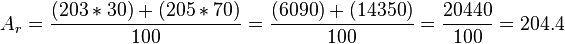# Relative atomic mass facts for kids

Kids Encyclopedia Facts
(Redirected from Atomic weight)

A relative atomic mass (also called atomic weight; symbol: Ar) is a measure of how heavy atoms are. It is the ratio of the average mass per atom of an element from a given sample to 1/12 the mass of a carbon-12 atom. In other words, a relative atomic mass tells you the number of times an average atom of an element from a given sample is heavier than one-twelfth of an atom of carbon-12. The word relative in relative atomic mass refers to this scaling relative to carbon-12. Relative atomic mass values are ratios; relative atomic mass is a dimensionless quantity. Relative atomic mass is the same as atomic weight, which is the older term.

The number of protons an atom has determines what element it is. However, most elements in nature consist of atoms with different numbers of neutrons. An atom of an element with a certain number of neutrons is called an isotope. For example, the element thallium has two common isotopes: thallium-203 and thallium-205. Both isotopes of thallium have 81 protons, but thallium-205 has 124 neutrons, 2 more than thallium-203, which has 122. Each isotope has its own mass, called its isotopic mass. A relative isotopic mass is the mass of an isotope relative to 1/12 the mass of a carbon-12 atom. The relative isotopic mass of an isotope is roughly the same as its mass number, which is the number of protons and neutrons in the nucleus. Like relative atomic mass values, relative isotopic mass values are ratios with no units.

We can find the relative atomic mass of a sample of an element by working out the abundance-weighted mean of the relative isotopic masses. For example, if a sample of thallium is made up of 30% thallium-203 and 70% thallium-205,$A_r = \frac{(203*30)+(205*70)}{100} = \frac{(6090)+(14350)}{100} = \frac{20440}{100} = 204.4$

Two samples of an element that consists of more than one isotope, collected from two widely spaced sources on Earth, are expected to have slightly different relative atomic masses. This is because the proportions of each isotope are slightly different at different locations.

A standard atomic weight is the mean value of relative atomic masses of a number of normal samples of the element. Standard atomic weight values are published at regular intervals by the Commission on Isotopic Abundances and Atomic Weights of the International Union of Pure and Applied Chemistry (IUPAC). The standard atomic weight for each element is on the periodic table.

Often, the term relative atomic mass is used to mean standard atomic weight. This is not quite correct, because relative atomic mass is a less specific term that refers to individual samples. Individual samples of an element could have a relative atomic mass different to the standard atomic weight for the element. For example, a sample from another planet could have a relative atomic mass very different to the standard Earth-based value.

Relative atomic mass is not the same as:

• atomic mass (symbol: ma), which is the mass of a single atom, commonly expressed in unified atomic mass units
• mass number (symbol: A), which is the sum of the number of protons and the number of neutrons in the nucleus of an atom
• atomic number (symbol: Z), which is the number of protons in the nucleus of an atom.Relative atomic mass Facts for Kids. Kiddle Encyclopedia.